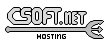Agar 1.7 Manual

SK(3)

# SYNOPSIS

```#include <agar/core.h>
#include <agar/sk.h>
```

# DESCRIPTION

The Agar SK class implements a general-purpose 2D "sketching" engine with dimensioning and geometrical constraint solving. Sketches define a set of elements and a set of relations between those elements.

Elements are organized in a tree (the same structure is used to described group and leaf nodes). Each element in the graph is associated with a transformation matrix. The major sketch elements are points, lines and arcs, but other types can be added through a class registration interface.

Relations represent geometric constraints affecting points, lines, circles, segments and arcs. The following constraints are implemented:
• Parallelism
• Incidence
• Perpendicularity
• Tangency
• Concentricity
• Colinearity
• Explicit distance
• Explicit angle

The SK_View(3) widget is commonly used to display and edit SK objects.

# INHERITANCE HIERARCHY

AG_Object(3)-> SK.

# INITIALIZATION

SK * SK_New (void *parent, const char *name)

The SK_New() function allocates, initializes, and attaches a SK object.

# NODE MANIPULATION

void SK_RegisterClass (SK_NodeOps *ops)

void SK_NodeInit (void *node, const SK_NodeOps *ops, Uint flags)

void * SK_NodeAdd (void *pnode, const SK_NodeOps *ops, Uint flags)

void SK_NodeAttach (void *pnode, void *node)

void SK_NodeDetach (void *pnode, void *node)

The SK_RegisterClass() function registers a new node class, described by the given SK_NodeOps structure.

The SK_NodeInit() function initializes the given SK_Node structure. It is usually invoked from node constructor functions. ops points to the SK_NodeOps structure which contains class information. The flags argument should be 0. The SK_NodeAdd() variant also allocates, initializes and attaches the node to a parent node.

The SK_NodeAttach() and SK_NodeDetach() functions attach/detach a node to/from a given parent.

# NODE TRANSFORMATIONS

These functions multiply a node's transformation matrix T with a translation, scaling or rotation matrix. They are only aliases for M_Matrix* functions, except that they accept a pointer to a node instead of a matrix.

void SK_Translatev (SK_Node *node, M_Vector3 v)

void SK_Translate2 (SK_Node *node, M_Real x, M_Real y)

void SK_Scalev (SK_Node *node, M_Vector3 v)

void SK_Rotatev (SK_Node *node, M_Real theta, M_Vector3 axis)

void SK_Rotatevd (SK_Node *node, M_Real degrees, M_Vector3 axis)

void SK_GetNodeTransform (void *node, M_Matrix44 *T)

The SK_Translate*() functions multiply T by a 2D translation matrix.
The SK_Scalev() function multiplies T by a 2D scaling matrix.
SK_Rotate*() multiply T by a 2D rotation matrix. Angles are given in radians, except for SK_Rotate*d() variants which accept angular arguments in degrees.
SK_Rotatev() generates a rotation of theta radians around axis. The SK_Rotate*() variants with the "d" suffix accept angles in degrees instead of radians.
The SK_GetNodeTransform() function returns a matrix which is the product of the transformation matrices of the given node and all of its parents.www.libAgar.org is © 2022 Julien Nadeau Carriere <vedge@csoft.net>.